Courses

# NCERT Exemplar (Part - 1) - Solid State Notes | EduRev

## JEE : NCERT Exemplar (Part - 1) - Solid State Notes | EduRev

The document NCERT Exemplar (Part - 1) - Solid State Notes | EduRev is a part of the JEE Course NEET Revision Notes.
All you need of JEE at this link: JEE

MULTIPLE CHOICE QUESTIONS - I

Q.1. Which of the following conditions favours the existence of a substance in the solid state?
(i) High temperature
(ii) Low temperature
(iii) High thermal energy
(iv) Weak cohesive forces

Ans. (ii)
Solution.
At low temperature substance exists in solid state due to decrease in molecular motion which leads to strong cohesive forces i.e., forces which hold the constituent particles together.

Q.2. Which of the following is not a characteristic of a crystalline solid?
(i) Definite and characteristic heat of fusion.
(ii) Isotropic nature.
(iii) A regular periodically repeated pattern of arrangement of constituent particles in the entire crystal.
(iv) A true solid

Ans. (ii)
Solution.

Anisotropy: Crystalline solids are anisotropic in nature, that is some of their physical properties like electrical resistance or refractive index show different values when measured along different directions in the same crystal. This arises from different arrangement of particles in different directions arrangement of particles along different directions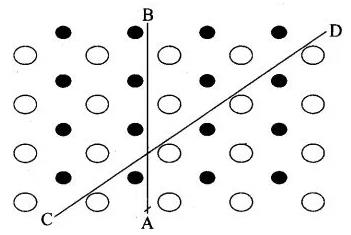Anisotropy in crystal is due to different arrangement of particles along different directions.

Isotropy: In case of amorphous substances, properties such as electrical conductivity, refractive index, thermal expansion, etc. are identical in all directions just as in case of gases or liquids. This property is called isotropy and the substances showing this property are called isotropic.

Q.3. Which of the following is an amorphous solid?
(i) Graphite (C)
(ii) Quartz glass (SiO2)
(iii) Chrome alum
(iv) Silicon carbide (SiC)
Ans. (ii)
Solution.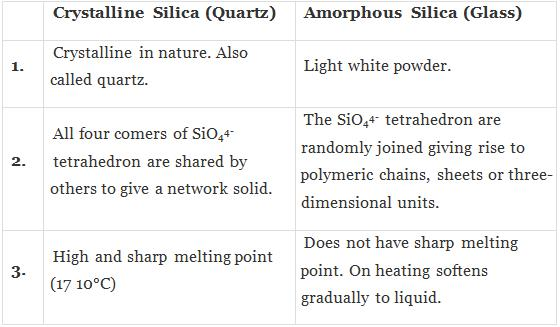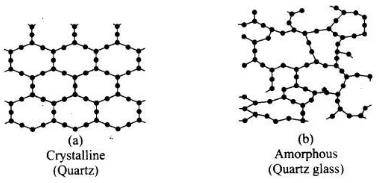Q.4. Which of the following arrangements shows schematic alignment of magnetic moments of antiferromagnetic substances?
(i)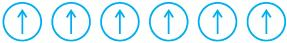(ii)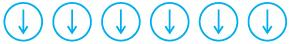(iii)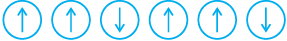(iv)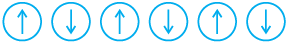Ans. (iv)
Solution.

The substances which have domain structure are oppositely oriented and cancel each other's magnetic field are known as antiferromagnetic substances.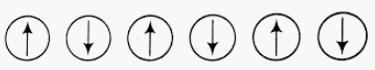Schematic arrangement of antiferromagnetic substance

Q.5. Which of the following is true about the value of refractive index of quartz glass?
(i) Same in all directions
(ii) Different in different directions
(iii) Cannot be measured
(iv) Always zero
Ans. (i)
Solution.

Since quartz glass is an amorphous solid having short range order of constitutents. Hence, value of refractive index is same in all directions, can be measured and not be equal to zero always.

Q.6. Which of the following statement is not true about amorphous solids?
(i) On heating they may become crystalline at certain temperature.
(ii) They may become crystalline on keeping for long time.
(iii) Amorphous solids can be moulded by heating.
(iv) They are anisotropic in nature.
Ans. (iv)
Solution.

Amorphous solids are isotropic in nature because it has no long range order and any physical property will be same in all directions. On the other hand, anisotropic nature is a characteristic feature of crystalline solid.

Q.7. The sharp melting point of crystalline solids is due to ___________.
(i) A regular arrangement of constituent particles observed over a short distance in the crystal lattice.
(ii) A regular arrangement of constituent particles observed over a long distance in the crystal lattice.
(iii) Same arrangement of constituent particles in different directions.
(iv) Different arrangement of constituent particles in different directions.
Ans. (ii)
Solution.

A solid is said to be crystalline if the various constituent structural units (atoms, ions or molecules) of which the solid is made, are arranged in a definite geometrical pattern within the solid.
The type of forces in crystalline solids are of long range order due to which they have sharp melting point.

Q.8. Iodine molecules are held in the crystals lattice by ____________.
(i) London forces
(ii) Dipole-dipole interactions
(iii) Covalent bonds
(iv) Coulombic forces
Ans. (i)
Solution.

Iodine molecules are a class of non-polar molecular solids in which constituent molecules are held together by weak London forces. These solids are soft and non-conductor of electricity.

Q.9. Which of the following is a network solid?
(i) SO2 (Solid)
(ii) I2
(iii) Diamond
(iv) H2O (Ice)
Ans. (iii)
Solution.

Diamond is a giant molecule in which constituent atoms are held together by covalent bond.
Hence, this is a network solid.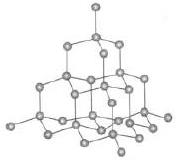Network structure of atom

Q.10. Which of the following solids is not an electrical conductor?
(A) Mg (s)
(B) TiO (s)
(C) I2(s)
(D) H2O (s)
(i) (A) only
(ii) (B) Only
(iii) (C) and (D)
(iv) (B), (C) and (D)
Ans. (iii)
Solution.

Iodine is a non-polar molecular solid in which iodine molecules are held together by London force or dispersion force. This is soft and non-conductor of electricity.
Water is a hydrogen bonded molecular solid in which H and 0 are held together by polar covalent bond and each water molecule held together by hydrogen bonding. Due to non-ionic nature, they are not electrical conductor.

Q.11. Which of the following is not the characteristic of ionic solids?
(i) Very low value of electrical conductivity in the molten state.
(ii) Brittle nature.
(iii) Very strong forces of interactions.
(iv) Anisotropic nature.
Ans. (i)
Solution.

Ionic solids easily dissociated into its ions in molten state and show high electrical conductivity. So, statement (i) is incorrect while ionic solids are anisotropic and brittle linked with very strong force of interactions.

Q.12. Graphite is a good conductor of electricity due to the presence of __________.
(i) Lone pair of electrons
(ii) Free valence electrons
(iii) Cations
(iv) Anions
Ans. (ii)
Solution.

Graphite is a good conductor of electricity due to presence of free valence electrons. In graphite, each carbon is sp2 hybridised having one free electron which makes graphite a good conductor of electricity.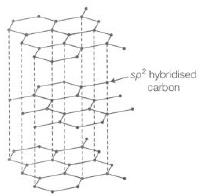Q.13. Which of the following oxides behaves as conductor or insulator depending upon temperature?
(i) TiO
(ii) SiO2
(iii) TiO3
(iv) MgO
Ans. (iii)
Solution.

Certain metal oxides like V02, VO, VO3 and TiO3 show metallic or insulating property depending upon temperature. As temperature varies metallic or insulating property varies. This is due to variation in energy gap between conduction band and valence band.

Q.14. Which of the following oxides shows electrical properties like metals?
(i) SiO2
(ii) MgO
(iii) SO2(s)
(iv) CrO2
Ans. (iv)
Solution.
CrO2, TiO and Re03 are some typical metal oxides which show electrical conductivity similar to metal. While SO2, MgO and SO2 are oxides of metal, semimetal and non-metal which do not show electrical properties.

Q.15. The lattice site in a pure crystal cannot be occupied by _________.
(i) Molecule
(ii) Ion
(iii) Electron
(iv) Atom
Ans. (iii)
Solution.

Pure crystals have constituents i.e., atoms or molecules or ions as lattice points which are arranged in fixed stoichiometric ratio. Electron can occupy the lattice site only when there is imperfection in solid and not in a pure crystal.
Hence, existence of free electrons are not possible, it is possible on in case of imperfection in solid.

Q.16. Graphite cannot be classified as __________.
(i) Conducting solid
(ii) Network solid
(iii) Covalent solid
(iv) Ionic solid

Ans. (iv)
Solution.

Constituent units of graphite are carbon atoms, held together by covalent bonding in 2D network structure. Thus, it is not an ionic solid.

Q.17. Cations are present in the interstitial sites in __________.
(i) Frenkel defect
(ii) Schottky defect
(iii) Vacancy defect
(iv) Metal deficiency defect
Ans. (i)
Solution.

When smaller ion (usually cation) is dislocated from its normal site in crystal and move to interstitial site is known as Frenkel defect as shown below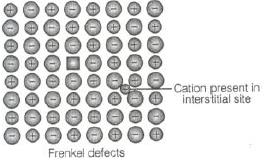Q.18. Schottky defect is observed in crystals when __________.
(i) Some cations move from their lattice site to interstitial sites.
(ii) Equal number of cations and anions are missing from the lattice.
(iii) Some lattice sites are occupied by electrons.
(iv) Some impurity is present in the lattice.

Ans. (ii)
Solution.

Schottky defect is observed in crystal when equal number of cations and anions are missing from the lattice.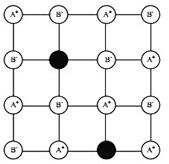Schottkey defect

When some cations move from their lattice site to interstitial site, this defect is known as Frenkel defect. When some impurity is present in crystal lattice it is known as impurity defect.
When lattice site is occupied by electron, this type of defect is known as metal excess defect.

Q.19. Which of the following is true about the charge acquired by p -type semiconductors?
(i) Positive
(ii) Neutral
(iii) Negative
(iv) Depends on concentration of p impurity
Ans. (ii)
Solution.

When group 13 elements are doped into the lattice of group 14 element, it creates a hole in amolecule but the molecule as a whole remains neutral. Thus, p -type semiconductor is neutral in nature.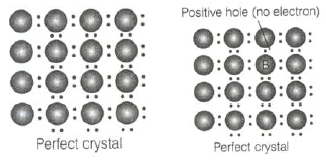Q.20. To get a n-type semiconductor from silicon, it should be doped with a substance with valence__________.
(i) 2
(ii) 1
(iii) 3
(iv) 5
Ans. (iv)
Solution.

Impurity of higher group is doped to get n-type semiconductor. Thus, silicon (valency = 4) should be doped with the element with valency equal to 5.

Q.21. The total number of tetrahedral voids in the face centred unit cell is __________.
(i) 6
(ii) 8
(iii) 10
(iv) 12
Ans. (ii)
Solution.

Fee unit cell contains 8 tetrahedral voids at centre of each 8 smaller cube of a unit cell as shown below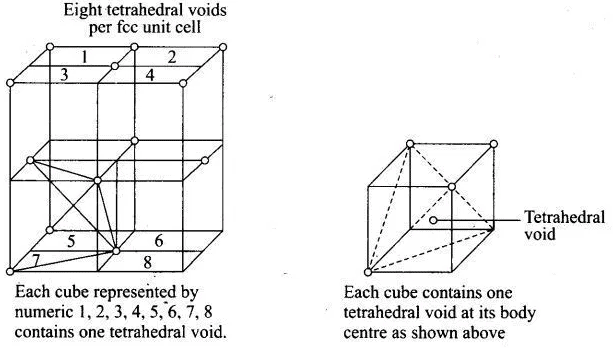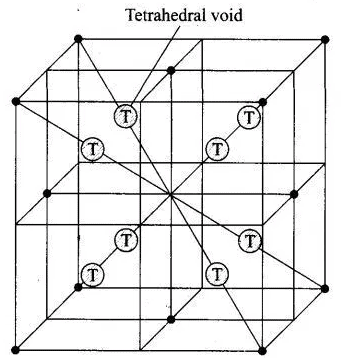Q.22. Which of the following point defects are shown by AgBr(s) crystals?
(A) Schottky defect
(B) Frenkel defect
(C) Metal excess defect
(D) Metal deficiency defect

(i) (A) and (B)
(ii) (C) and (D)
(iii) (A) and (C)
(iv) (B) and (D)

Ans. (i)
Solution.

AgBr shows both Schottky and Frenkel defects. In AgBr, both Ag+ and Br ions are absent from the lattice causing Schottky defect. However, Ag+ ions are mobile so they have a tendency to move aside the lattice and trapped in interstitial site, hence cause Frenkel defect.

Q.23. In which pair most efficient packing is present?
(i) hcp and bcc
(ii) hcp and ccp
(iii) bcc and ccp
(iv) bcc and simple cubic cell
Ans. (ii)
Solution.

Packing efficiency: It is the percentage of total filled space by particles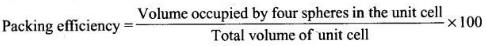Since, packing efficiency for hep or ccp is calculated to be 74% which is maximum among all type of crystals.

Q.24. The percentage of empty space in a body centred cubic arrangement is ________.
(i) 74
(ii) 68
(iii) 32
(iv) 26
Ans. (iii)
Solution.

Packing efficiency for bcc arrangement is 68% which represents total filled space in the unit cell. Hence, empty space in a body centered arrangement is 100 – 68 = 32%

Q.25. Which of the following statement is not true about the hexagonal close packing?
(i) The coordination number is 12.
(ii) It has 74% packing efficiency.
(iii) Tetrahedral voids of the second layer are covered by the spheres of the third layer.
(iv) In this arrangement spheres of the fourth layer are exactly aligned with those of the first layer.
Ans. (iv)
Solution.

Hexagonal close packing can be arranged by two layers
A and B one over another which can be diagrammatically represented as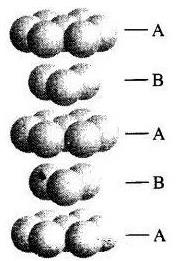Hexagonal Close Packing

Here, we can see easily that 1st layer and 4th layer are not exactly aligned. Thus, statement (d) is not correct while other statements (a), (b) and (c) are true.

Q.26. In which of the following structures coordination number for cations and anions in the packed structure will be same?
(i) Cl ion form fcc lattice and Na+ ions occupy all octahedral voids of the unit cell.
(ii) Ca2+ ions form fcc lattice and F – ions occupy all the eight tetrahedral voids of the unit cell.
(iii) O2– ions form fcc lattice and Na+ ions occupy all the eight tetrahedral voids of the unit cell.
(iv) S2– ions form fcc lattice and Zn2+ ions go into alternate tetrahedral voids of the unit cell.
Ans. (i)
Solution.

NaCl crystals have rock salt structure having fee lattice in which Cl ions are present at fee lattice points and face centre and Na+ occupies all the octahedral voids of given unit cell.
Where, coordination number of Na+ = 6
Coordination number of Cl= 6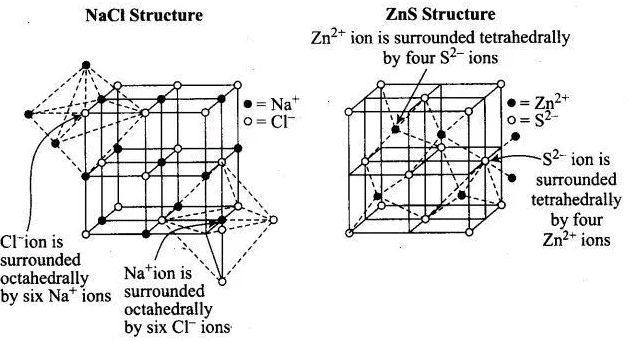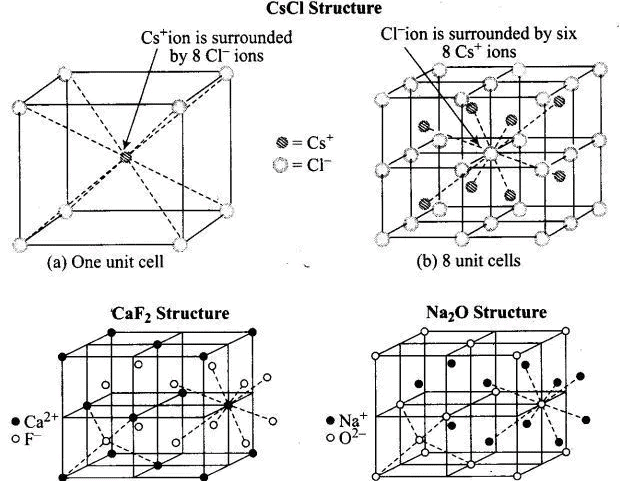Q.27. What is the coordination number in a square close packed structure in two dimensions?
(i) 2
(ii) 3
(iii) 4
(iv) 6
Ans. (iii)
Solution.

Coordination number in a square closed packed structure in two dimensions is equal to 4 is shown as: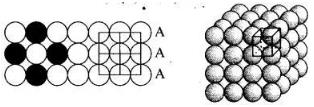Q.28. Which kind of defects are introduced by doping?
(i) Dislocation defect
(ii) Schottky defect
(iii) Frenkel defects
(iv) Electronic defects
Ans. (iv)
Solution.

When electron rich or electron deficient impurity is added to a perfect crystal, it introduces electronic defect in them.

Q.29. Silicon doped with electron-rich impurity forms  ________.
(i) p-type semiconductor
(ii) n-type semiconductor
(iii) intrinsic semiconductor
(iv) insulator
Ans. (ii)
Solution.

Silicon has four valence electrons. If it is doped with an electron rich impurity, the extra electron becomes delocalised and increases the conductivity. Since the increase in conductivity is due to negatively charged electron, hence it is called n-type semiconductor.

Q.30. Which of the following statements is not true?
(i) Paramagnetic substances are weakly attracted by magnetic field.
(ii) Ferromagnetic substances cannot be magnetized permanently.
(iii) The domains in antiferromagnetic substances are oppositely oriented with respect to each other.
(iv) Pairing of electrons cancels their magnetic moment in the diamagnetic substances.
Ans. (ii)
Solution.

Ferromagnetic species are strongly attracted in the magnetic field and can be permanently magnetised.
Hence, choice (ii) is the correct answer while other three choices are correct.

Q.31. Which of the following is not true about the ionic solids?
(i) Bigger ions form the close packed structure.
(ii) Smaller ions occupy either the tetrahedral or the octahedral voids depending upon their size.
(iii) Occupation of all the voids is not necessary.
(iv) The fraction of octahedral or tetrahedral voids occupied depends upon the radii of the ions occupying the voids.
Ans. (iv)
Solution.

The fraction of octahedral or tetrahedral voids occupied depends upon the radii of the ions present at the lattice points. As we know the radii of octahedral or tetrahedral void is related to radii of atoms (r) as Radius of octahedral void (R0) = 0.414 r.
Radius of tetrahedral void (R1) = 0.225 r  Where, r = radius of bigger atom involved.

Q.32. A ferromagnetic substance becomes a permanent magnet when it is placed in a magnetic field becuase ________.
(i) All the domains get oriented in the direction of magnetic field.
(ii) All the domains get oriented in the direction opposite to the direction of magnetic field.
(iii) Domains get oriented randomly.
(iv) Domains are not affected by magnetic field.

Ans. (i)
Solution.

Ferromagnetic solids can be permanently magnetised and then all the domains get oriented in the direction of applied magnetic field.

Q.33. The correct order of the packing efficiency in different types of unit cells is ________.
(i) fcc < bcc < simple cubic
(ii) fcc > bcc > simple cubic
(iii) fcc < bcc > simple cubic
(iv) bcc < fcc > simple cubic
Ans. (ii)
Solution.

Packing efficiency in different types of unit cells can be tabulated as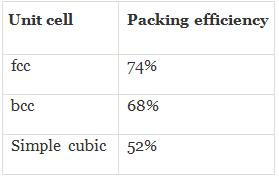Hence, correct order is fee (74%) > bee (68%) > simple cubic (52%).

Q.34. Which of the following defects is also known as dislocation defect?
(i) Frenkel defect
(ii) Schottky defect
(iii) Non-stoichiometric defect
(iv) Simple interstitial defect
Ans. (i)
Solution.

In Frenkel defect, some cations occupy interstitial site and hence it is also called dislocation defect.

Q.35. In the cubic close packing, the unit cell has ________.
(i) 4 tetrahedral voids each of which is shared by four adjacent unit cells.
(ii) 4 tetrahedral voids within the unit cell.
(iii) 8 tetrahedral voids each of the which is shared by four adjacent unit cells.
(iv) 8 tetrahedral voids within the unit cells.
Ans. (iv)
Solution.

In the cubic close packing the unit cell has 8 tetrahedral voids within it and are located at each eight smaller cube of the unit cell

Q.36. The edge lengths of the unit cells in terms of the radius of spheres constituting fcc, bcc and simple cubic unit cell are respectively________.
(i)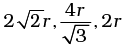(ii)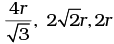(iii)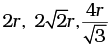(iv)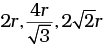Ans. (i)
Solution.

Note: Distance between two atoms is always measured from their centres
(i) If the crystal lattice consists of SCC, the atom which is present at the comers touch each other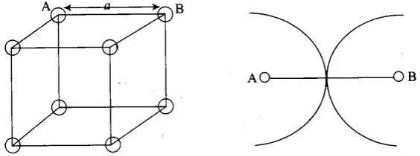(ii) In case of FCC, atom present at the comer and the centre of the face touch each other.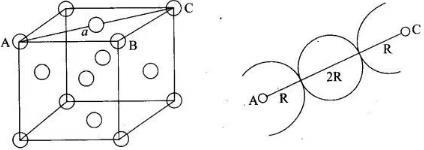(iii) In case of BCC atom present at the corner and center of the body touch each other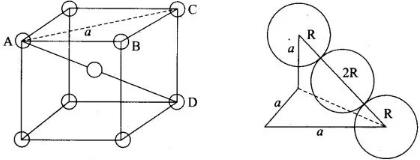Edge length for different types of unit cells can be tabulated as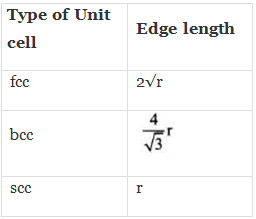Q.37. Which of the following represents correct order of conductivity in solids?
(i) κmetals >> κinsulators < κsemiconductors
(ii) κmetals<< κinsulators < κsemiconductors
(iii) κmetals < κsemiconductors > κinsulators = zero
(iv) κmetals < κsemiconductors > κinsulators ≠ zero
Ans. (i)
Solution.

Conductivity of metal, insulator and semiconductors can be represented in the term of k (Kappa) which depends upon energy gap between valence band and conduction band.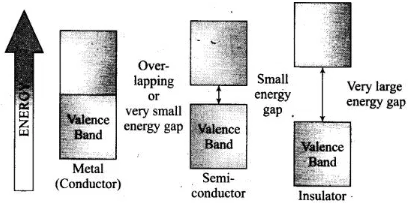Valence and Conductance bands in metals, semiconductors and insulators.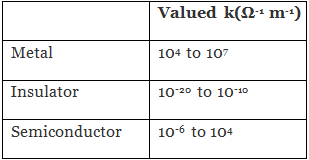MULTIPLE CHOICE QUESTIONS - II

Note : In the following questions two or more options may be correct.
Q.38. Which of the following is not true about the voids formed in 3 dimensional hexagonal close packed structure?
(i) A tetrahedral void is formed when a sphere of the second layer is present above triangular void in the first layer.
(ii) All the triangular voids are not covered by the spheres of the second layer.
(iii) Tetrahedral voids are formed when the triangular voids in the second layer lie above the triangular voids in the first layer and the triangular shapes of these voids do not overlap.
(iv) Octahedral voids are formed when the triangular voids in the second layer exactly overlap with similar voids in the first layer.
Ans. (iii, iv)
Solution.
Tetrahedral voids are formed when the triangular void in the second layer lie exactly above the triangular voids in the first layer and the triangular shape of these voids oppositely overlap.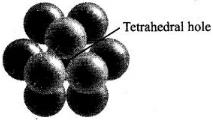Octahedral voids are formed when triangular void of second layer is not exactly overlap with similar void in first layer.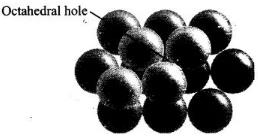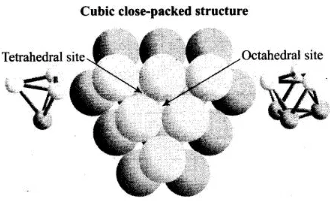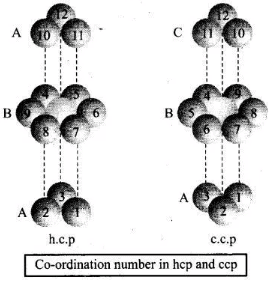Q.39. The value of magnetic moment is zero in the case of antiferromagnetic substances because the domains ________.
(i) Get oriented in the direction of the applied magnetic field.
(ii) Get oriented opposite to the direction of the applied magnetic field.
(iii) Are oppositely oriented with respect to each other without the application of magnetic field.
(iv) Cancel out each other’s magnetic moment.
Ans. (iii, iv)
Solution.

In the case of antiferromagnetic substances, the magnetic moment becomes zero because the domains are oppositely oriented with respect to each other without the application of magnetic field which cancel out each other.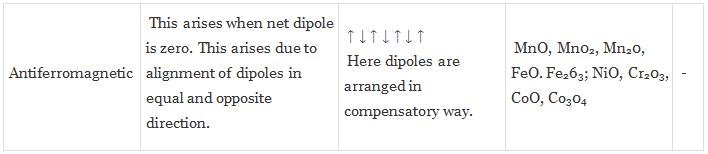Q.40. Which of the following statements are not true?
(i) Vacancy defect results in a decrease in the density of the substance.
(ii) Interstitial defects results in an increase in the density of the substance.
(iii) Impurity defect has no effect on the density of the substance.
(iv) Frankel defect results in an increase in the density of the substance.
Ans. (iii, iv)
Solution.

Statements (iii) and (iv) can be correctly written as (iii) Impurity defect
changes the density of substance as impurity has different than the ion present on perfect crystal e.g., When SrCl2 is added to the NaCl crystal, it causes impurity defect, (iv) Frenkel defect results neither decrease nor increase in density of substance.

Q.41. Which of the following statements are true about metals?
(i) Valence band overlaps with conduction band.
(ii) The gap between valence band and conduction band is negligible.
(iii) The gap between valence band and conduction band cannot be determined.
(iv) Valence band may remain partially filled.
Ans. (i, ii, iv)
Solution.

In metal, valence band overlap with conduction band. The gap between valence band and conduction band is negligible and valence band may remain partially filled.

Q.42. Under the influence of electric field, which of the following statements is true about the movement of electrons and holes in a p-type semi conductor?
(i) Electron will move towards the positively charged plate through electron holes.
(ii) Holes will appear to be moving towards the negatively charged plate.
(iii) Both electrons and holes appear to move towards the positively charged plate.
(iv) Movement of electrons is not related to the movement of holes.
Ans. (i, ii)
Solution.

In p-type semiconductor, the conductivity is due to existence of hole. When electric field is applied to p-type semiconductor hole starts moving towards negatively charged plate and electron towards positively charged plate.
Flow of holes in p-type semiconductors Hole .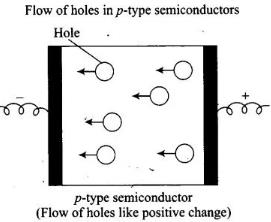Q.43. Which of the following statements are true about semiconductors?
(i) Silicon doped with electron rich impurity is a p-type semiconductor.
(ii) Silicon doped with an electron rich impurity is an n-type semiconductor.
(iii) Delocalised electrons increase the conductivity of doped silicon.
(iv) An electron vacancy increases the conductivity of n-type semiconductor.

Ans. (ii, iii)
Solution.

Silicon (valence electron – 4) doped with electron rich impurity is an n-type semiconductor due to extra electron and the delocalised electrons increase the conductivity of doped silicon.

Q.44. An excess of potassium ions makes KCl crystals appear violet or lilac in colour since ________.
(i) Some of the anionic sites are occupied by an unpaired electron.
(ii) Some of the anionic sites are occupied by a pair of electrons.
(iii) There are vacancies at some anionic sites.
(iv) F-centres are created which impart colour to the crystals.
Ans. (i, iv)
Solution.

When KC1 is heated in vapour of K, some of the Cl” leave their lattice site and create anion vacancies. This chloride ion wants to combine with K vapour to form potassium chloride. For doing so K atom loses electrons form K ions. This released electron diffuses into the crystal to get entrapped in the anion vacancy called F-centre. When visible light falls on the crystal, this entrapped electron gains energy, goes to the higher level when it comes back to the ground state, energy is released in the form of light.

Q.45. The number of tetrahedral voids per unit cell in NaCl crystal is ________.
(i) 4
(ii) 8
(iii) Twice the number of octahedral voids.
(iv) Four times the number of octahedral voids.
Ans. (ii, iii)
Solution.

NaCl has fee arrangement of CF ions. Thus,
Number of CF ions in packing per unit cell = 4
Number of tetrahedral voids = 2 x No. of particles present in close packing
= 2 × 4 = 8
Number of tetrahedral voids = 2 x No. of octahedral voids

Q.46. Amorphous solid can also be called ________.
(i) Pseudo solids
(ii) True solids
(iii) Super cooled liquids
(iv) Super cooled solids
Ans. (i, iii)
Solution.

Amorphous solid has short range order which has a tendency to flow very slowly. Hence, it is also known as pseudo solids or super cooled liquids. Glass panes fixed to windows or doors of old buildings are invariably observed to be thicker at bottom than at the top. These are examples of amorphous solids.

Q.47. A perfect crystal of silicon (Fig. 1.1) is doped with some elements as given in the options. Which of these options show n-type semiconductors?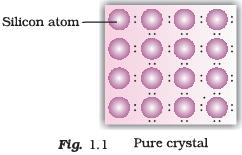(i)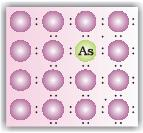(ii)(iii)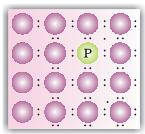(iv)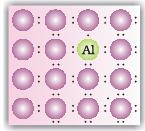Ans. (i, iii)
Solution.
When group 15 elements are doped into a perfect crystal, it leads to the formation of n-type semiconductor.
Here, in (a) as (group 15, period 3) is doped to perfect Si-crystal and in (c) as (group 15, period 2) is doped to perfect Si-crystal.

Q.48. Which of the following statements are correct?
(i) Ferrimagnetic substances lose ferrimagnetism on heating and become paramagnetic.
(ii) Ferrimagnetic substances do not lose ferrimagnetism on heating and remain ferrimagnetic.
(iii) Antiferromagnetic substances have domain structures similar to ferromagnetic substances and their magnetic moments are not cancelled by each other.
(iv) In ferromagnetic substances all the domains get oriented in the direction of magnetic field and remain as such even after removing magnetic field.

Ans. (i, iv)
Solution.

Ferrimagnetic substances lose ferrimagnetism on heating and become paramagnetic. In ferromagnetic substance, domains are aligned in parallel and antiparallel direction in unequal numbers.
In ferromagnetic substances, all the domains get oriented in the direction of magnetic field and remain as such even after removing magnetic field.

Q.49. Which of the following features are not shown by quartz glass?
(i) This is a crystalline solid.
(ii) Refractive index is same in all the directions.
(iii) This has definite heat of fusion.
(iv) This is also called super cooled liquid.

Ans. (i, iii)
Solution.

Quartz glass is an amorphous solid so it has not definite heat of fusion. This is due to short range order of molecule while quartz glass is also known as super cooled liquid and isotropic in nature.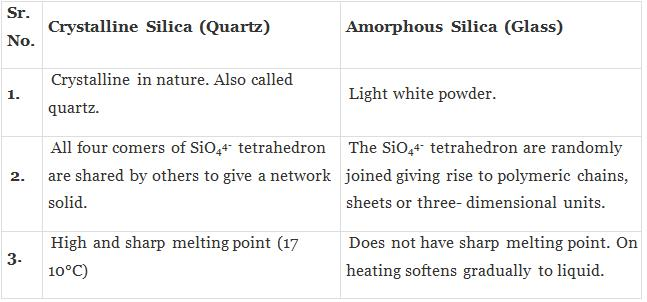Q.50. Which of the following cannot be regarded as molecular solid?
(i) SiC (Silicon carbide)
(ii) AlN
(iii) Diamond
(iv) I2
Ans. (i, ii, iii)
Solution.

SiC, AIN and diamond are examples of network solid as they have three dimensional structure while, I2 is a molecular solid, because such solid particles are held together by dipole-dipole interactions. SiC and AIN are interstitial solids.

Q.51. In which of the following arrangements octahedral voids are formed?
(i) hcp
(ii) bcc
(iii) simple cubic
(iv) fcc
Ans. (i, iv)
Solution.

In hcp and fee arrangement, octahedral voids are formed. In fcc, the octahedral voids are observed at edge and centre of cube while in bcc and simple cubic, no any octahedral voids are observed. In bcc, cubic voids formed.

Q.52. Frenkel defect is also known as ________.
(i) Stoichiometric defect
(ii) Dislocation defect
(iii) Impurity defect
(iv) Non-stoichometric defect
Ans. (i, ii)
Solution.

In Frenkel defect, dislocation of cations takes place and there is no change in stoichiometry of the crystal.

Q.53. Which of the following defects decrease the density?
(i) Interstitial defect
(ii) Vacancy defect
(iii) Frankel defect
(iv) Schottky defect
Ans. (ii, iv)
Solution.

Vacancy and Schottky defect which lead to decrease the density both are the types of a stoichiometric defect. In case of Frenkel defect and interstitial defect, there is no change in density of substance.

Offer running on EduRev: Apply code STAYHOME200 to get INR 200 off on our premium plan EduRev Infinity!

,

,

,

,

,

,

,

,

,

,

,

,

,

,

,

,

,

,

,

,

,

;